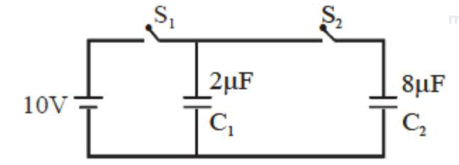# A 2 muF capacitor CQuestion:

A $2 \mu \mathrm{F}$ capacitor $\mathrm{C}_{1}$ is first charged to a potential difference of $10 \mathrm{~V}$ using a battery. Then the battery is removed and the capacitor is connected to an uncharged capacitor $\mathrm{C}_{2}$ of $8 \mu \mathrm{F}$.

The charge in $\mathrm{C}_{2}$ on equilibrium condition is $--\mu \mathrm{C}$. (Round off to the Nearest Integer)Solution:

(16)

$20=\left(\mathrm{C}_{1}+\mathrm{C}_{2}\right) \mathrm{V} \Rightarrow \mathrm{V}=2$ volt

$\mathrm{Q}_{2}=\mathrm{C}_{2} \mathrm{~V}=16 \mu \mathrm{C}$

$=16$